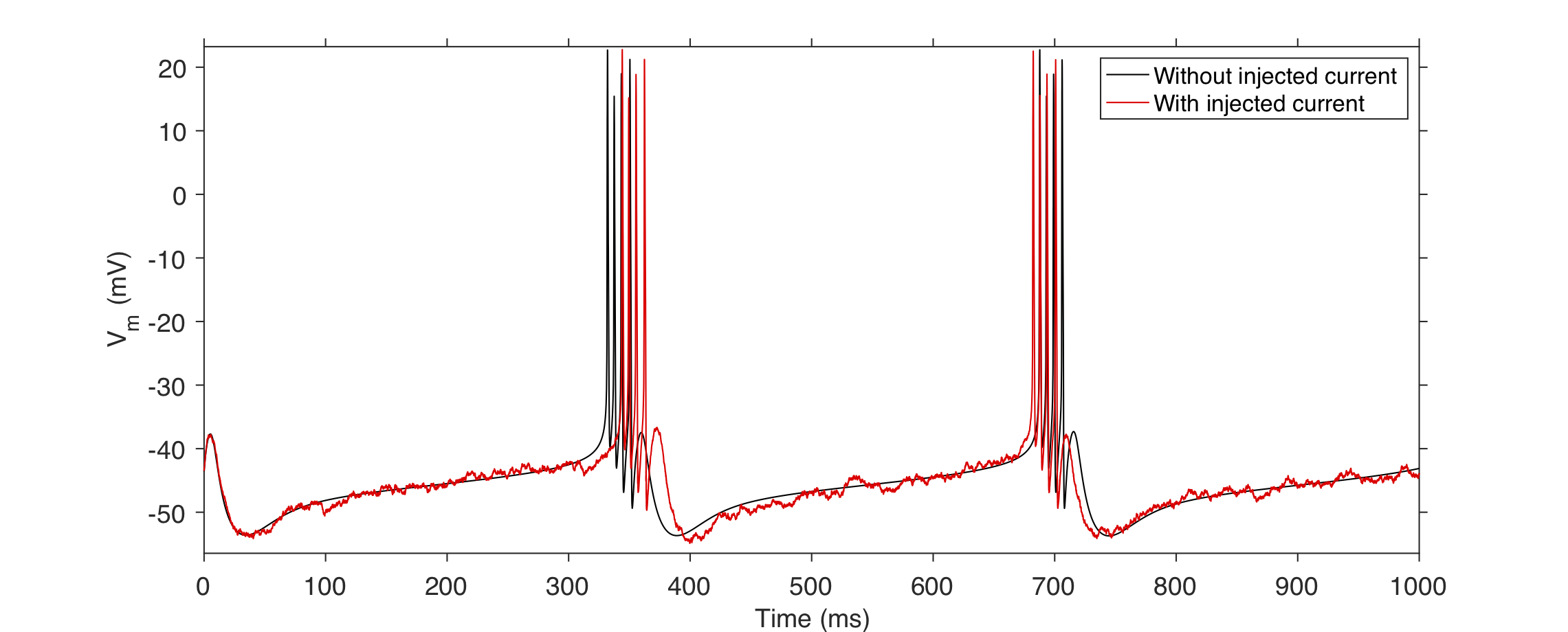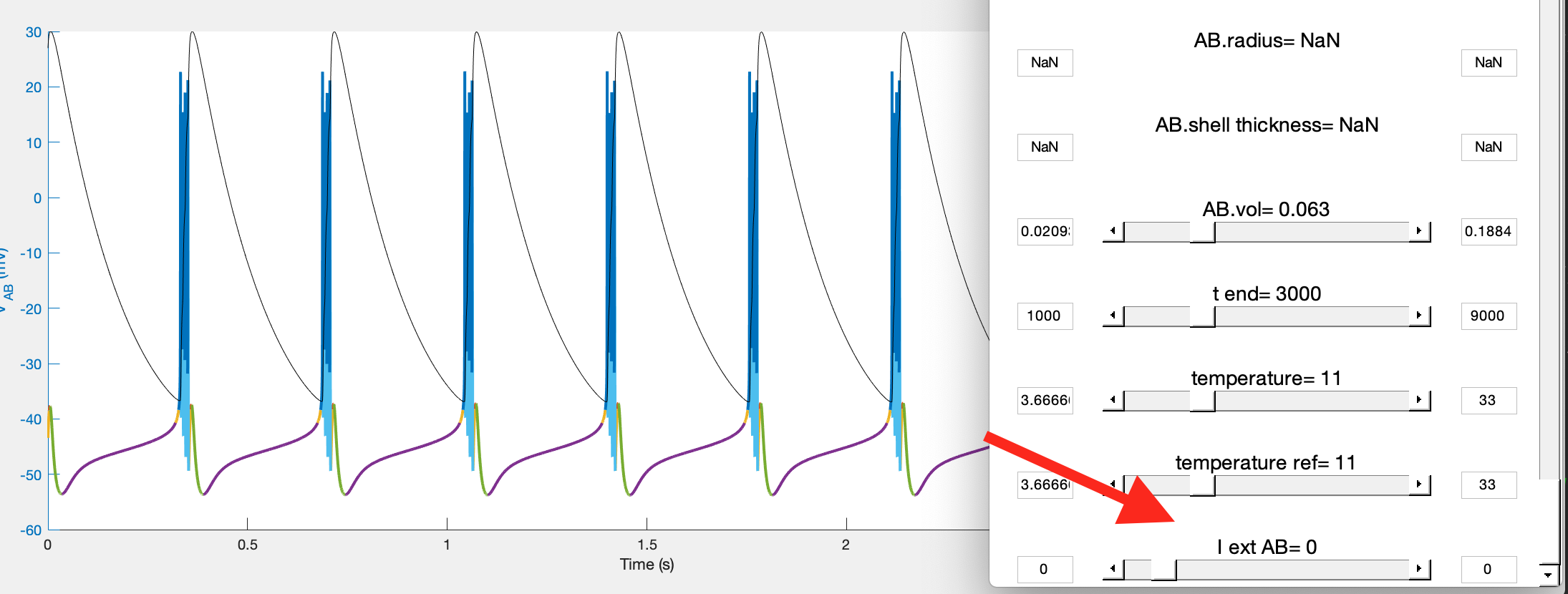# Inject current

This document describes how to inject current in a compartment in xolotl.

## Limitations¶

• You cannot simultaneously inject current and voltage clamp a model
• Current injection is not supported when using the Runge-Kutta solver

## How to inject current into a compartment¶

### Inject a constant current into a single compartment model¶

The simplest way to inject current into a compartment is to set the I_ext property in xolotl. Assuming you have a single compartment model called x,

x.I_ext = .1; % nA


will inject 1 nA of current into the compartment. That's it.

### Inject a constant current in a multi-compartment model¶

The size of I_ext in the second dimension is the number of compartments. Therefore, to inject a constant current into the compartment you want, set that element of I_ext. For example, if x is a model with three compartments,

x.I_ext = [0 1 0];


will inject 1 nA into the second compartment, but nothing into the other two.

### Inject a time-varying current into a compartment¶

xolotl supports injecting arbitrary, time-varying currents into a compartment, as long as they are determined in advance. In this case, I_ext must be a matrix whose size in the first dimension should be equal to x.t_end/x.sim_dt

Warning

A common error is tying to set I_ext to a vector whose length is x.t_end/x.dt. Note that x.dt and x.sim_dt can be different.

For example, if we want to inject a random current into a neuron model, we can do something like this:

x = xolotl.examples.neurons.BurstingNeuron;
x.I_ext = randn(x.t_end/x.sim_dt,1);Warning

If you specify a time-varying x.I_ext, and then change x.t_end or x.sim_dt, I_ext may be overwritten if it is of an incompatible size.

## Manipulating models while injecting current¶

### Injecting constant current¶

By default, manipulating a model using x.manipulate allows you to vary the amount of constant current applied to the neuron:This also means that if you specify a time-varying current, that I_ext is overwritten with a constant value when you call x.manipulate()

### Injecting time-varying current¶

To manipulate a model while injecting a custom, time-varying current, set this preference:

x.pref.use_I_ext = false;


Now, your custom I_ext will be used, and sliders for I_ext will not be shown when manipulate is called.